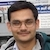# NAEST 2017 Screening Test: Equation of ContinuityBy

In screening round of National Anveshika Experimental Skills Test ( NAEST 2017 ), a question was asked on the flow of fluids. The water is flowing through a pipe of circular cross-section. It comes out of the pipe horizontally and travels in a parabolic path hitting the ground at some distance. When end of the pipe is pressed, the water hits the ground farther away.

## Question from NAEST 2017

Choose the correct option(s) from the following:

1. The cross-section area of the pipe does not change when its opening is pressed.
2. The cross-section area of the pipe decreases when its opening is pressed.
3. The cross-section area of the pipe increases when its opening is pressed.
4. The speed of water coming out of the pipe decreases when pipe is pressed.
5. The speed of water coming out of the pipe increases when pipe is pressed.
6. The range of projectile path increases when speed of water is decreased.
7. The range of projectile path decreases when speed of water is decreased.

Solution: When opening of the pipe is pressed, the water travels more distance due to increase in in the flow speed. This can be explained using a result from the geometry and the equation of continuity.

The shape of pipe's cross-section changes from circular to elliptical when its opening is pressed. In this process, the perimeter of the pipe remains same but its cross-section area decreases. It can be shown that area of the circle is maximum for a given perimeter. Thus, cross-section area of the opening decreases from $A_1$ to $A_2$ when it is pressed.Let $v_0$ and $A_0$ be the flow speed and cross-sectional area at the inlet. The flow speed is $v_1$ when opening is circular and it is $v_2$ when opening is elliptical. Apply continuity equation in two cases to get \begin{align} A_0 v_0 =A_1 v_1 \\ A_0 v_0 = A_2 v_2 \end{align} which gives \begin{align} v_2&=v_1(A_1/A_2) \\ &> v_1\;\; (\text{because}\, A_1>A_2) \end{align} Note that equation of continuity is a statement of mass conservation for incompressible blow.Let height of the pipe from the ground be $h$. We assume that water stream takes a parabolic path similar to the path taken by a particle projected horizontally. The time taken by a fluid particle to reach the ground is \begin{align} t=\sqrt{2h/g} \end{align}The horizontal distance travelled by the stream in two cases are \begin{align} x_1&=v_1 t =v_1\sqrt{2h/g}, \\ x_2&=v_2 t =(A_1/A_2) v_1\sqrt{2h/g}, \\ & > x_1 \;\; (\text{because}\, A_1>A_2) \end{align} Thus, stream travels more horizontal distance when pipe's opening is pressed.